# Isosceles Triangle Theorem

Anderson Gomes Da Silva, Jeff Calareso
• Author
Anderson Gomes Da Silva

Anderson holds a Bachelor's and Master's Degrees (both in Mathematics) from the Fluminense Federal University and the Pontifical Catholic University of Rio de Janeiro, respectively. He was a Teaching Assistant at the University of Delaware (UD) for two and a half years, leading discussion and laboratory sessions of Calculus I, II and III. In the Winter of 2021 he was the sole instructor for one of the Calculus I sections at UD.

• Instructor
Jeff Calareso

Jeff teaches high school English, math and other subjects. He has a master's degree in writing and literature.

Study how to prove congruent isosceles triangles. Using the isosceles triangle theorem and its converse, learn how to prove that a triangle is isosceles. Updated: 12/18/2021

Show

## Congruent Isosceles Triangles

A triangle is a polygon with three sides, three vertices, and three interior angles. Triangles can be classified according to their sides and interior angles. In particular, a triangle is said to be isosceles when at least two of their sides are congruent, that is, their lengths are the same. As a consequence of that, the angles in the base are congruent as well. This is going to be shown later. So, congruency in isosceles triangles refers to the congruence of a pair of sides or the congruence of the base angles. It is also true that if two angles in a triangle are congruent, then the sides that are opposite to them are congruent too. Both statements made in this section are going to be proven in this lesson.

## If, Then

Here's an isosceles triangle.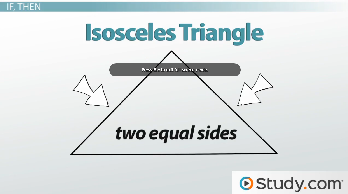We know it's an isosceles triangle because it has two equal sides. That's the definition of an isosceles triangle. But if it's an isosceles triangle, what else can we prove?

Geometry is full of these if, then statements, just like life. Some of these are simple. If a shape has four equal sides and four right angles, then it's a square. That's like saying if you go swimming, you're going to get wet. There's really no ambiguity there.

With an isosceles triangle, there are some 'if, then' statements that seem logical, but we need to test them to be sure. It's like saying if you make guacamole, then it's going to be awesome. We can't be sure of this until you make some guacamole, right? Then we need to test it by sampling some. And maybe we aren't so sure with just one taste. Why don't we try a whole bowl? Then we'll know for sure.

Anyway, an isosceles triangle has parts we can label. We call the equal sides of isosceles triangles the legs. The third side is called the base. The angles across from the legs are called the base angles.

We know our triangle has equal sides, or legs, but let's try to prove a theorem. There's a theorem that states that if two sides of a triangle are congruent, then the angles opposite these sides are also congruent. Is this 'if, then' statement true?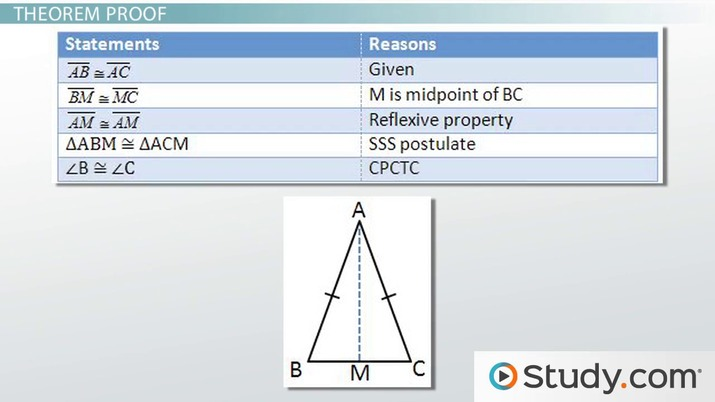An error occurred trying to load this video.

Try refreshing the page, or contact customer support.

Coming up next: Angles Formed by a Transversal

### You're on a roll. Keep up the good work!

Replay
Your next lesson will play in 10 seconds
• 0:01 If, Then
• 1:23 Theorem Proof
• 2:32 Converse Proof
• 4:24 Lesson Summary
Save Save

Want to watch this again later?

Timeline
Autoplay
Autoplay
Speed Speed

## What Is The Isosceles Triangle Theorem?

A statement of the form "if p, then q" has as its inverse the statement "if q, then p". The isosceles triangle theorem and the base angles theorem are converses of each other. The first starts with having two congruent sides as a given fact and ends with proving that there are two congruent angles using congruence of triangles, in which case, corresponding elements of congruent triangles are congruent. The latter starts with two congruent angles as a given and ends with the proof that the opposite sides to the angles are congruent.

## Isosceles Triangle Proof

Theorem: the base angles of an isosceles triangle are congruent.

Proof: Consider an isosceles triangle {eq}ABC {/eq} with {eq}AB {/eq} congruent to segment {eq}AC {/eq}. Figure 1 depicts this and the congruence between the segments is indicated by the decoration with one dash on each one of them.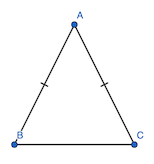The goal is to show that the base angles, that is {eq}\angle~ABC {/eq} and {eq}\angle~ACB {/eq} are congruent. For this end, draw the bisector of angle {eq}BAC {/eq}. Recall that a bisector is a ray that divides an angle into two congruent ones. This procedure gives Figure 2, with {eq}AD {/eq} being the bisector and angles {eq}BAD {/eq} and {eq}DAC {/eq} being congruent. This is indicated by the similar decoration on both angles.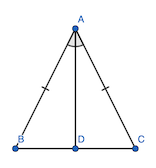The bisector divides the original triangle {eq}ABC {/eq} into two triangles, which are {eq}\triangle~BAD {/eq} and {eq}\triangle~CAD {/eq}. Claim: the two triangles are congruent. Indeed, {eq}AB~\cong~AC {/eq} (because the triangle {eq}ABC {/eq} is isosceles). Besides {eq}AD {/eq} is a side common to both triangles. Finally, {eq}\angle~BAD~\cong~\angle~CAD {/eq} because segment {eq}AD {/eq} was simply constructed to divide {eq}\angle~BAC {/eq} into two equal angles. The conclusion is that by the case of congruence of triangles side-angle-side (abbreviated as SAS), the two triangles are congruent as desired. (Remark: when using this case of congruence of triangles, keep in mind that the angle has to be the one formed by the two sides involved in the process, which was the case here). As a consequence, the homologous pairs of elements from both triangles are congruent. In particular, {eq}\angle~ABD~\cong~\angle~ACD {/eq}. This ends the proof that the base angles of an isosceles triangle are congruent.

## The Converse of Isosceles Triangle Theorem

It is not always the case that the converse of a statement that is true is also true. In the context of this lesson, however, the converse of the statement proven in the previous section is also true. This fact is going to be proven in this section.

Theorem: Let {eq}ABC {/eq} be a triangle with {eq}\angle~ABC~\cong~\angle~ACB {/eq}. Then, {eq}\triangle~ABC {/eq} is isosceles with {eq}AB {/eq} being congruent to {eq}AC {/eq}.

## Theorem Proof

Let's prove the theorem. Here's triangle ABC.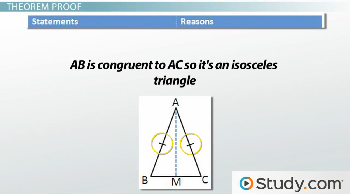We're given that AB is congruent to AC. It's 'isosceles-iness' is therefore established. We want to prove that angle B is congruent to angle C.

First, let's state what we know. AB is congruent to AC. That's given. Now, let's add a midpoint on BC and call it M and a line from A to M. This is a median line.

We can then state that BM is congruent to MC. Next, let's state that AM is congruent to AM because of the reflexive property, also known as, well, it's the same line.

AB and AC, BM and MC, and AM and AM. That's three sides of the two triangles formed when we added the median. So triangle ABM is congruent to triangle ACM because of the side-side-side postulate.

That allows us to state that angle B is congruent to angle C because corresponding parts of congruent triangles are congruent, or CPCTC.

So our theorem is true! That's almost as satisfying as figuring out that your guacamole is awesome.

## Converse Proof

We proved our theorem, but what about its converse? The converse of an 'if, then' statement is tricky.

To unlock this lesson you must be a Study.com Member.

Video Transcript

## If, Then

Here's an isosceles triangle.We know it's an isosceles triangle because it has two equal sides. That's the definition of an isosceles triangle. But if it's an isosceles triangle, what else can we prove?

Geometry is full of these if, then statements, just like life. Some of these are simple. If a shape has four equal sides and four right angles, then it's a square. That's like saying if you go swimming, you're going to get wet. There's really no ambiguity there.

With an isosceles triangle, there are some 'if, then' statements that seem logical, but we need to test them to be sure. It's like saying if you make guacamole, then it's going to be awesome. We can't be sure of this until you make some guacamole, right? Then we need to test it by sampling some. And maybe we aren't so sure with just one taste. Why don't we try a whole bowl? Then we'll know for sure.

Anyway, an isosceles triangle has parts we can label. We call the equal sides of isosceles triangles the legs. The third side is called the base. The angles across from the legs are called the base angles.

We know our triangle has equal sides, or legs, but let's try to prove a theorem. There's a theorem that states that if two sides of a triangle are congruent, then the angles opposite these sides are also congruent. Is this 'if, then' statement true?

## Theorem Proof

Let's prove the theorem. Here's triangle ABC.We're given that AB is congruent to AC. It's 'isosceles-iness' is therefore established. We want to prove that angle B is congruent to angle C.

First, let's state what we know. AB is congruent to AC. That's given. Now, let's add a midpoint on BC and call it M and a line from A to M. This is a median line.

We can then state that BM is congruent to MC. Next, let's state that AM is congruent to AM because of the reflexive property, also known as, well, it's the same line.

AB and AC, BM and MC, and AM and AM. That's three sides of the two triangles formed when we added the median. So triangle ABM is congruent to triangle ACM because of the side-side-side postulate.

That allows us to state that angle B is congruent to angle C because corresponding parts of congruent triangles are congruent, or CPCTC.

So our theorem is true! That's almost as satisfying as figuring out that your guacamole is awesome.

## Converse Proof

We proved our theorem, but what about its converse? The converse of an 'if, then' statement is tricky.

To unlock this lesson you must be a Study.com Member.

#### Are all isosceles triangles congruent?

No, not all isosceles triangles are congruent. For instance, a triangle with three 60 degrees interior angles is not congruent to one with two 45 degrees angles.

#### How do you find the missing side of an isosceles triangle?

To find the missing side of an isosceles triangle it is necessary to know the measure of one angle and one side. It is given by x = y sin a/sin b, where a is the angle opposite to x and b, the one opposite to y.

### Register to view this lesson

Are you a student or a teacher?